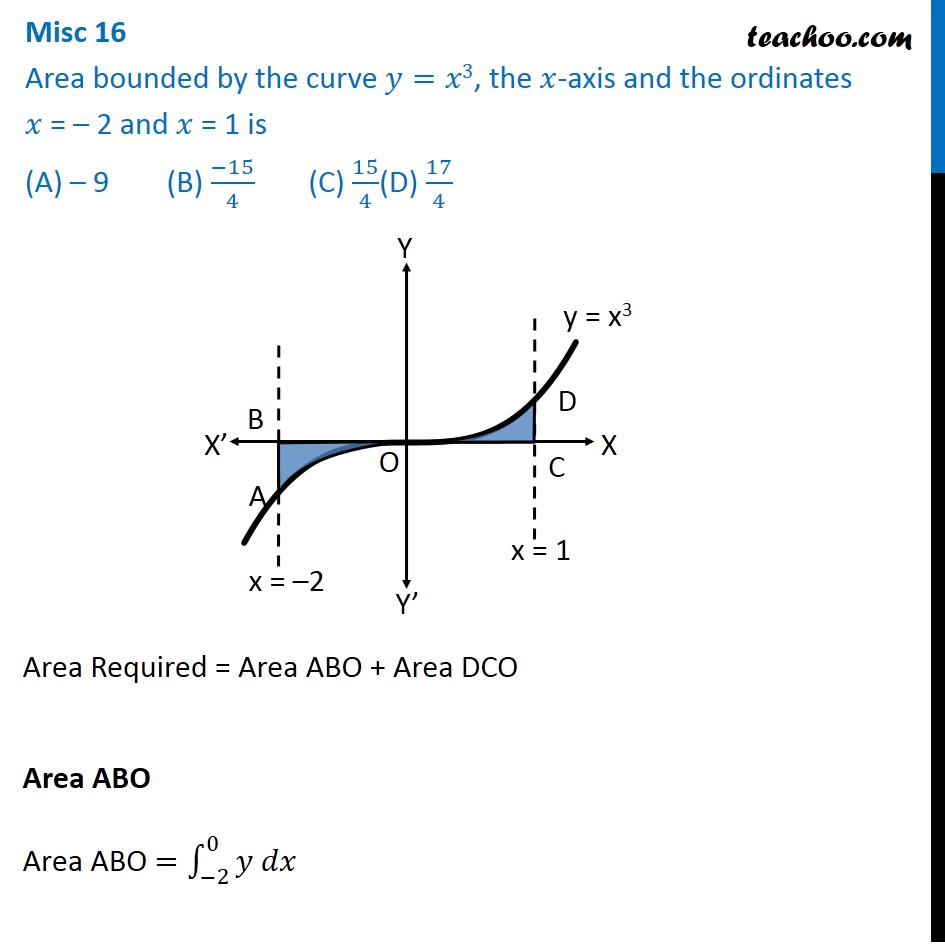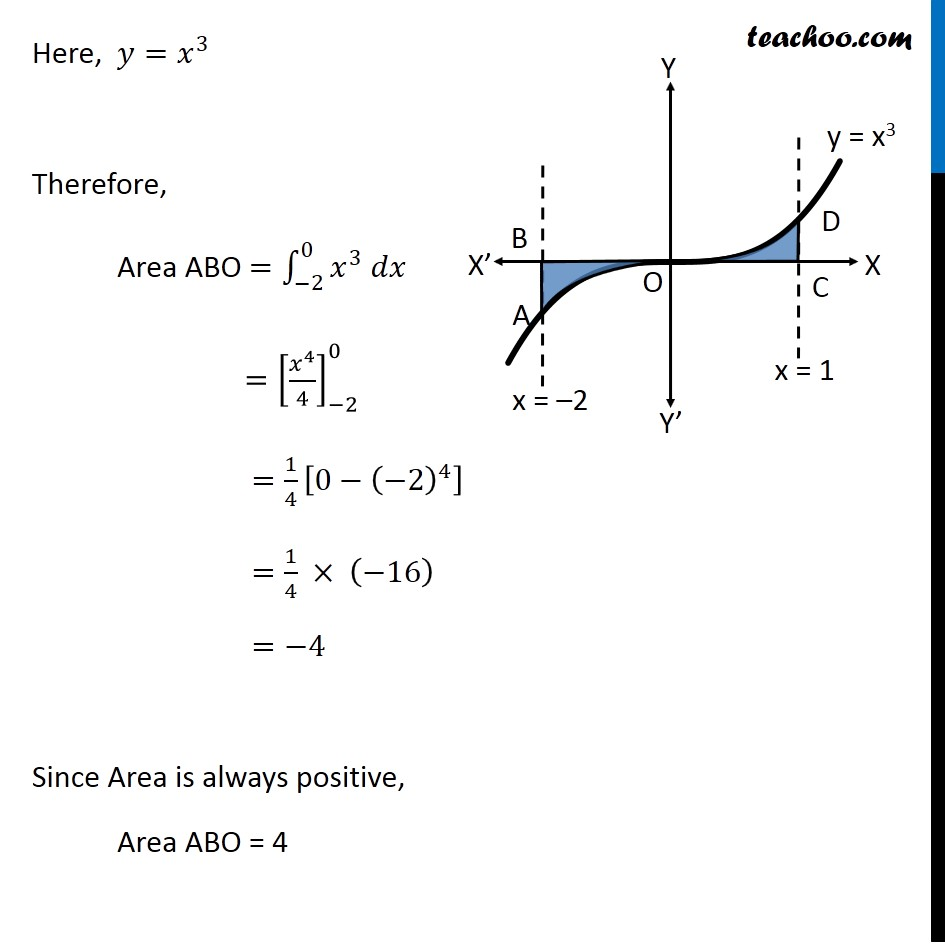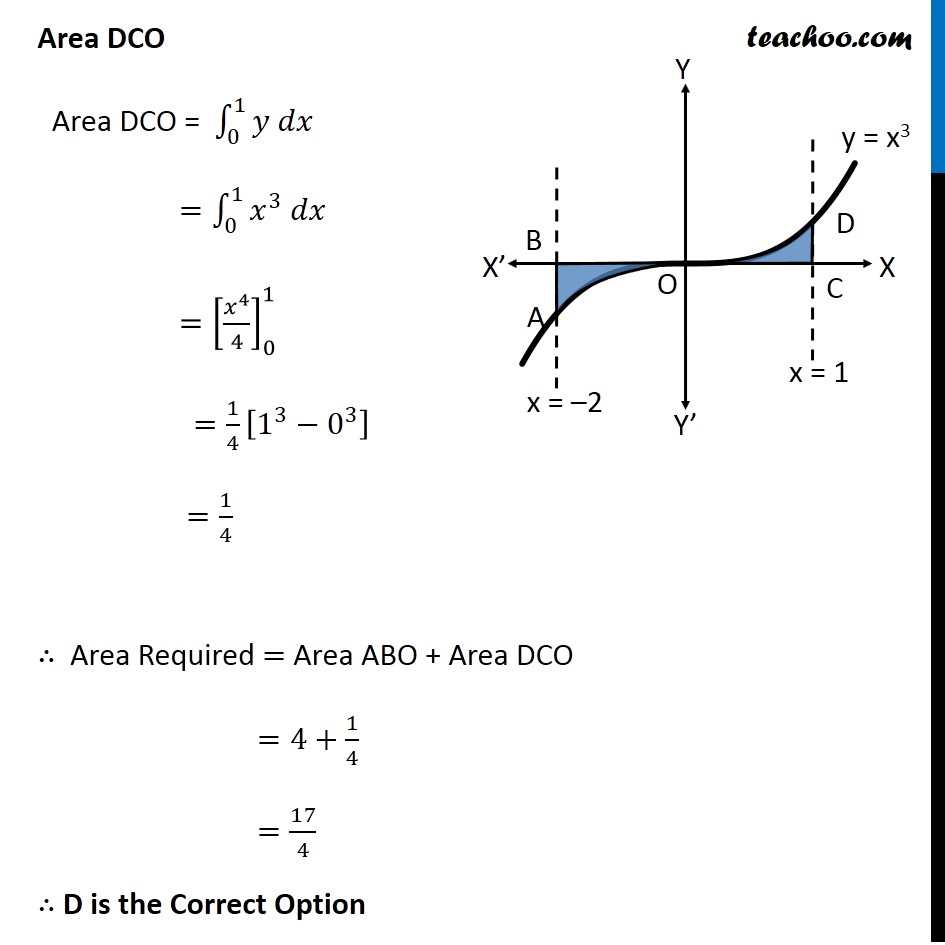Miscellaneous

Chapter 8 Class 12 Application of Integrals
Serial order wiseLearn in your speed, with individual attention - Teachoo Maths 1-on-1 Class

### Transcript

Misc 16 Area bounded by the curve 𝑦=𝑥3, the 𝑥-axis and the ordinates 𝑥 = – 2 and 𝑥 = 1 is (A) – 9 (B) (−15)/4 (C) 15/4 (D) 17/4 Area Required = Area ABO + Area DCO Area ABO Area ABO =∫_(−2)^0▒〖𝑦 𝑑𝑥〗 Here, 𝑦=𝑥^3 Therefore, Area ABO =∫_(−2)^0▒〖𝑥^3 𝑑𝑥〗 〖=[𝑥^4/4]〗_(−2)^0 =1/4 [0−(−2)^4 ] =1/4 × (−16) =−4 Since Area is always positive, Area ABO = 4 Area DCO Area DCO = ∫_0^1▒〖𝑦 𝑑𝑥〗 =∫_0^1▒〖𝑥^3 𝑑𝑥〗 =[𝑥^4/4]_0^1 =1/4 [1^3−0^3 ] =1/4 ∴ Area Required = Area ABO + Area DCO =4+1/4 =17/4 ∴ D is the Correct Option